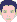## (4): Volume Percent, Mass Percent

support
WUFI UserPosts: 33
Joined: Thu Mar 03, 2005 2:35 am -1100

### (4): Volume Percent, Mass Percent

WUFI gives me the water content of the simulated wall in units of kg/m³ or in volume percent. However, I need the result in mass percent. How do I convert the results?

WUFI usually gives the water content as "water density", i.e. how many kg of water are in one m³ of building material.
A result given in volume percent tells you how many m³ of water are in one m³ of building material (expressed as percentage).
A result given in mass percent tells you how many kg of water are in one kg of dry building material (expressed as percentage). Please note that the water content in mass percent may easily exceed 100% if the dry material has low density.

With
m_W : mass of the water in the component
r_W : density of water (= 1000 kg/m³)
V_W : volume of the water in the component
m_C : mass of the component
r_C : density of the (dry) component
V_C : volume of the component

we have

water content as expressed by WUFI:
u = m_W / V_C [kg/m³]

water content expressed in volume percent:
u_v = V_W / V_C * 100
= (m_W / r_W) / V_C * 100
= (m_W / V_C) / r_W * 100
= u * 100 / r_W
= u * 100 / 1000
= u / 10

water content expressed in mass percent:
u_m = m_W / m_C * 100
= m_W / (r_C * V_C ) * 100
= (m_W / V_C) * (100 / r_C)
= u * (100 / r_C)
= u / (r_C / 100)

So you get the water content in volume percent if you divide the WUFI result [kg/m³] by 10.
You get the water content in mass percent if you divide the WUFI result by (density of the building component / 100).